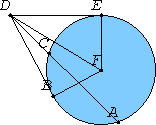# Proposition 37

If a point is taken outside a circle and from the point there fall on the circle two straight lines, if one of them cuts the circle, and the other falls on it, and if further the rectangle contained by the whole of the straight line which cuts the circle and the straight line intercepted on it outside between the point and the convex circumference equals the square on the straight line which falls on the circle, then the straight line which falls on it touches the circle.

Let a point D be taken outside the circle ABC, from D let the two straight lines DCA and DB fall on the circle ACB, let DCA cut the circle and DB fall on it, and let the rectangle AD by DC equal the square on DB.

I say that DB touches the circle ABC.Draw DE touching ABC. Take the center F of the circle ABC, and join FE, FB, and FD.

III.18

Thus the angle FED is right.

III.36

Now, since DE touches the circle ABC, and DCA cuts it, the rectangle AD by DC equals the square on DE.

But the rectangle AD by DC was also equal to the square on DB, therefore the square on DE equals the square on DB. Therefore DE equals DB.

I.8

And FE equals FB, therefore the two sides DE and EF equal the two sides DB and BF, and FD is the common base of the triangles, therefore the angle DEF equals the angle DBF.

But the angle DEF is right, therefore the angle DBF is also right.

III.16 Cor

And FB produced is a diameter, and the straight line drawn at right angles to the diameter of a circle, from its end, touches the circle, therefore DB touches the circle.

Similarly this can be proved to be the case even if the center is on AC.

Therefore if a point is taken outside a circle and from the point there fall on the circle two straight lines, if one of them cuts the circle, and the other falls on it, and if further the rectangle contained by the whole of the straight line which cuts the circle and the straight line intercepted on it outside between the point and the convex circumference equals the square on the straight line which falls on the circle, then the straight line which falls on it touches the circle.

Q.E.D.

## Guide

This proposition is used in IV.10.